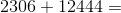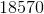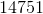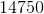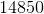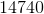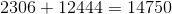## Example Questions

### Example Question #78 : Integers

The sum of seven consecutive even integers is 0.

Column A: The product of the seven integers

Column B: 2

The two quantities are equal.

Column A is greater.

The relationship cannot be determined from the given information.

Column B is greater.

Column B is greater.

Explanation:

For the sum of 7 consecutive even integers to be zero, the only sequence possible is –6, –4, –2, 0, 2, 4, 6. This can be determined algebraically by assigning the lowest number in the sequence to be “y” and adding 2 for each consecutive even integer, and then setting this equal to zero.

y, y + 2, y + 4, y + 6 . . .

The product of any number and 0 is 0.

Therefore, column B must be greater.

### Example Question #79 : Integersand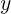are both even whole numbers.

What is a possible solution for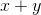?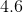Explanation:

Ifandare both even whole numbers, then their addition must be an even whole number as well. Althoughis an even number, it is not a whole number and could therefore not be a solution. This means the only possible solution would be.

### Example Question #80 : Integers

If John buys twenty-two apples on Monday, and thirty-four bananas on Tuesday, how many pieces of fruit does John have?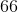Explanation: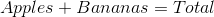Plugging in the values given we arrive at the total fruit John has: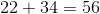A good note about adding even numbers--any even numbers--is that if you add two even numbers, their sum will ALWAYS be an even number.

### Example Question #1 : Even / Odd Numbers

Choose the answer below which best solves the following problem: